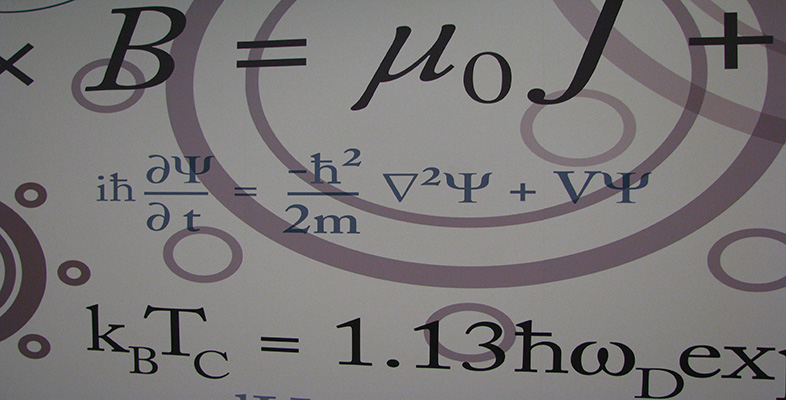Number systems

This free course is available to start right now. Review the full course description and key learning outcomes and create an account and enrol if you want a free statement of participation.

Free course

2.11 Further exercises

Exercise 28

Let z1 = 2 + 3i and z2 = 1 − 4i. Find z1 + z2, z1z2, z1z2,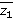,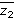, z1/z2 and 1/z1.

Solution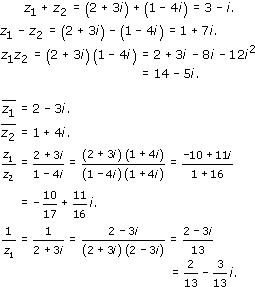Exercise 29

Draw a diagram showing each of the following complex numbers in the complex plane, and express them in polar form, using principal arguments.

• (a)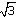i

• (b)  −5i

• (c)  −2 − 2i

Solution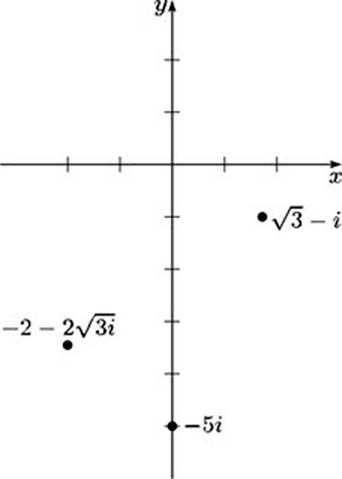• (a)  Let z = x + iy =i, so x =and y = − 1. Then z = r(cos θ + i sin θ), where

•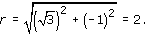• Also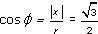. So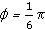, and z lies in the fourth quadrant, so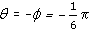.

• Hence the polar form ofi in terms of the principal argument is

•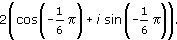• (b)  Let z = x + iy = −5i, so x = 0 and y = −5. Then z = r(cos θ + i sin θ), where

•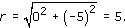• Also z lies on the negative half of the imaginary axis, so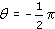.

• Hence the polar form of −5i in terms of the principal argument is

•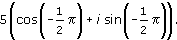• (c)  Let z = x + iy = −2 − 2i, so x = −2 and y = −2. Then z = r(cos θ + i sin θ), where

•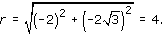• Also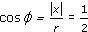. So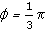, and z lies in the third quadrant, so

•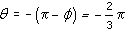.

• Hence the polar form of −2 − 2i in terms of the principal argument is

•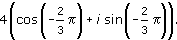Exercise 30

Express each of the following complex numbers in Cartesian form.

• (a)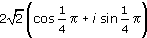• (b)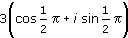• (c)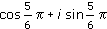Solution

• (a)  The required form is x + iy, where

•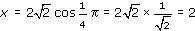• and

•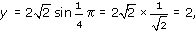• so the Cartesian form is 2 + 2i.

• (b)  The required form is x + iy, where

•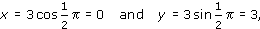• so the Cartesian form is 3i.

• (c)  The required form is x + iy, where

•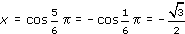• and

•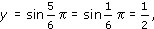• so the Cartesian form is

•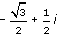Exercise 31

Let z1 =i, z2 = −5i and z3 = −2 −2i. Use the solution to Exercise 29 to determine the following complex numbers in polar form in terms of the principal argument.

• (a)  z1z2z3

• (b)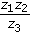Solution

From the solution to Exercise 29, we have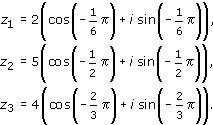• (a)  Hence

•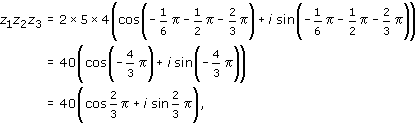• using the principal argument.

• (b)

•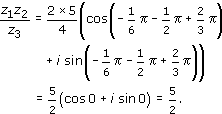Exercise 32

Solve the equation z5 = −32, leaving your answers in polar form.

Solution

Let z = r(cos θ + i sin θ).

Then, since −32 = 32(cos+ i sin), we have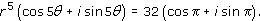Hence r = 2 and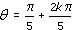for any integer k, and the five solutions of z5 = −32 are given by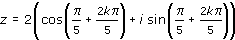for k = 0, 1, 2, 3, 4.

Hence the solutions are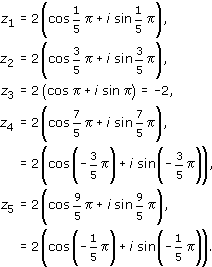Exercise 33

Solve the equation z3 + z2z + 15 = 0, given that one solution is an integer.

Solution

The integer solution must be a factor of the constant term 15,

so it must be one of ± 1, ± 3, ± 5, ± 15.

Testing these, we find z = −3 is a root, since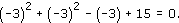Hence z + 3 is a factor, and we find that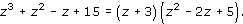The solutions of z2 −2z + 5 = 0 are given by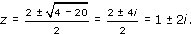Hence the solutions of z3 + z2z + 15 = 0 are z = −3, z =1 + 2i and z = 1 − 2i.

Exercise 34

Determine a polynomial of degree 4 whose roots are 3, −2, 2 − i and 2 + i.

Solution

A suitable polynomial is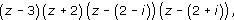that is,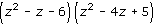or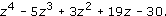Exercise 35

Use de Moivre's Theorem to obtain formulas for cos 6θ and sin 6θ in terms of cos θ and sin θ.

Solution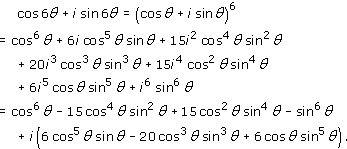Hence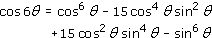and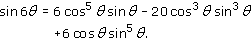Exercise 36

Use the definition of ez to express the following complex numbers in Cartesian form.

• (a)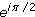• (b)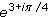• (c)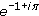Solution

• (a)

•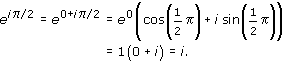• (b)

•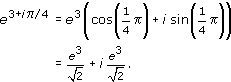• (c)= e−1(cos+ isin) = −e−1.

M208_6